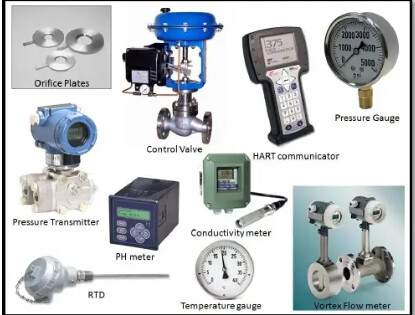# Instrumentation DefinitionProcess: The physical system that we are trying to measure or control. Some examples are a water filtration system, a system for pouring molten metal, a steam boiler, a unit for refining oil, and a unit for making electricity.

Process Variable, or PV: The precise quantity being measured in a process. Pressure, level, temperature, flow, electrical conductivity, pH, location, speed, and vibration are some examples.

Setpoint, or SP: The target value at which we want to keep the process variable.

Primary Sensing Element, or PSE: A device that senses the process variable directly and converts the sensed quantity into an analog representation (electrical voltage, current, resistance; mechanical force, motion, etc.). Thermocouple, thermistor, bourdon tube, microphone, potentiometer, electrochemical cell, and accelerometer are some examples.

Transducer: A piece of equipment that processes and/or transforms a standard instrumentation signal into another standard instrumentation signal. Switches between two different voltages; also known as a “relay” and “converter.” Here are some examples: an I/P converter (which takes a 4-20 Ma electric signal and changes it to a 3-15 PSI pneumatic signal) or a P/I converter (which takes a 3-15 PSI pneumatic signal and converts it to a 4-20 Ma electric signal).

Transmitter: An instrument that takes a signal from a primary sensing element (PSE) and converts it into a standard instrumentation signal (e.g., 3-15 PSI air pressure, 4-20 mA DC electric current, Fieldbus digital signal packet, etc.) that can be sent to an indicating device, a controlling device, or both.

LRV and URV: Process measurement values that are 0 and 100 percent of a transmitter’s measured range. For example, if a temperature transmitter is calibrated to measure a range of temperature starting at 300 degrees Celsius and ending at 500 degrees Celsius, 300 degrees would be the LRV and 500 degrees would be the URV.

Zero and Span: Possible substitutes for “zero” and “one hundred percent” in describing an instrument’s zero and one hundred percent ranges, respectively. An instrument’s “span” is the difference between its minimum and maximum values, while its “zero” is the starting point of its range (equal to LRV). As an illustration, if a temperature transmitter is set up to measure temperatures between 300 and 500 degrees Celsius, its zero temperature and span temperature settings would be 300 and 200 degrees, respectively.

Controller: A device that receives a process variable (PV) signal from a primary sensing element (PSE) or transmitter, compares that signal to the process variable’s desired value (known as the setpoint), and determines the proper output signal value to be sent to a final control element (FCE), such as an electric motor or control valve.

Final Control Element, or FCE: A device that receives a signal from a controller and uses it to alter the process directly. Variable-speed electric motor, control valve, and electric heater are some examples.

Manipulated Variable, or MV: A controller’s output signal. This signal manipulates the final process control element.